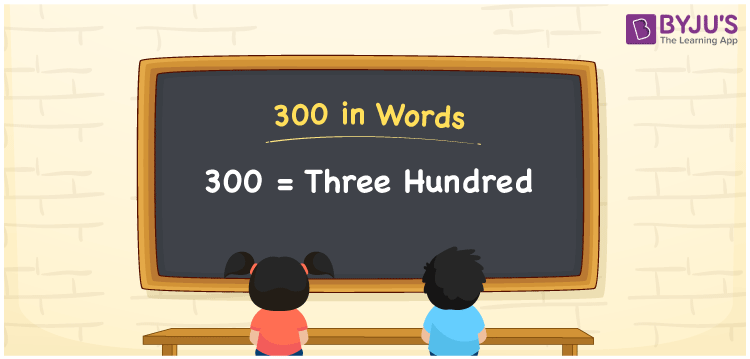# 300 in Words

The number 300 in words is written as Three hundred. The number in words is frequently written using place values, such as ones, tens, hundreds and so on. Thus, to write the number 300 in words, the place value chart is very helpful. For example, if you purchased a shirt that cost Rs. 300, you can write “I purchased a shirt that cost Rs. Three hundred”. Here, we are going to discuss the spelling of 300 in English and also how to write the numerical name of 300 using the place value chart in this article.

 300 in Words: Three hundred Three hundred in Numerical Form: 300

## 300 in English Words## How to Write 300 in Words?

To write the number 300 in words, first, identify the place value of each digit of the number 300. The following is the place value chart for the number 300.

 Hundreds Tens Ones 3 0 0

Using the place value chart, the expanded form of 300 is written as follows:

= 3 × Hundred × 0 × Ten + 0 × One

= 3 × 100 + 0 × 10 + 0 × 1.

= 300

= Three hundred

Therefore, 300 in words is Three hundred.

The natural number 300 is found after 299, but just before the number 301.

300 in words – Three hundred

Is 300 an odd number? – No

Is 300 an even number? – Yes

Is 300 a perfect square number? – No

Is 300 a perfect cube number? – No

Is 300 a prime number? – No

Is 300 a composite number? – Yes

## Frequently Asked Questions on 300 in Words

Q1

### How to write 300 in words?

300 in words is three hundred.

Q2

### Simplify 500 – 200, and express in words.

Simplifying 500 – 200, we get 300. Hence, 300 in words is three hundred.

Q3

### How to write three hundred in numbers?

Three hundred in numbers is 300.